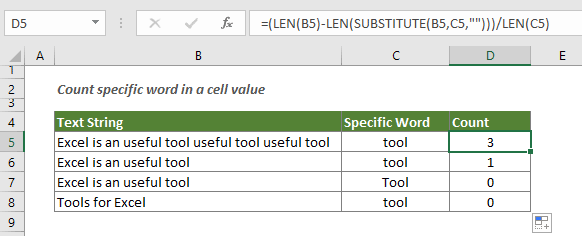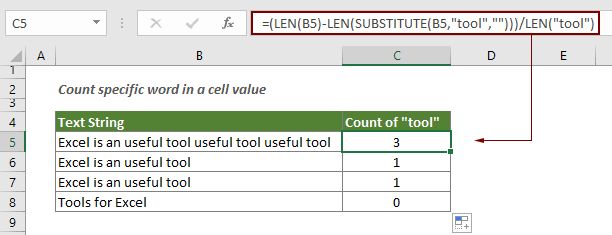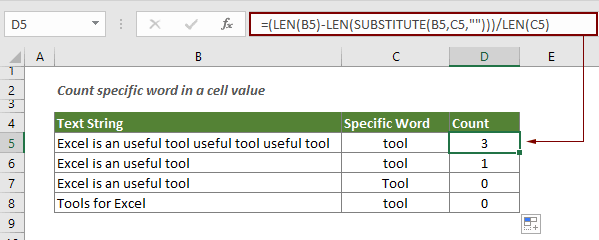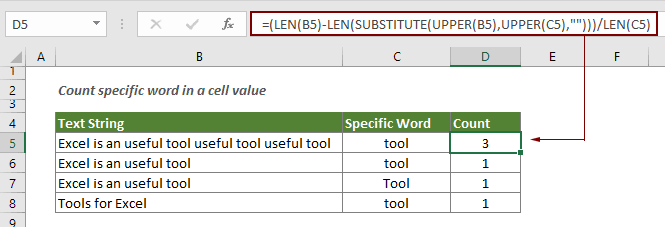## Count specific words in a cell in Excel#### How to count specific words in a cell in Excel?

Generic formula

=(LEN(cell_ref)-LEN(SUBSTITUTE(cell_ref, word,"")))/LEN(word)

Arguments

Cell_ref: The cell contains the text string you will count specific word inside.

Word: The specific word you want to count. It can be:
1. The word enclosed in quotation marks;
2. Or a reference to a cell containing the word.

How to use this formula?

Here is the step-by-step guide to help you apply the formula in Excel.

For example, you want to count number of times the word “tool” appears in cell B5, please do as follows.

Select a blank cell, copy the below formula into it to get the result immediately.

=(LEN(B5)-LEN(SUBSTITUTE(B5,"tool","")))/LEN("tool")Tips: Instead of directly typing the word in the formula, you can type the word in a certain cell, and then reference this cell in the formula. See the below formula and screenshot:
=(LEN(B5)-LEN(SUBSTITUTE(B5,C5,"")))/LEN(C5)Notes:

• 1. Please change the cell B5 and word “tool” to your own needs;
• 2. If the word is not found, it will return 0;
• 3. The formula is case-sensitive;
• 4. If you want to count specific word in a cell and ignore the case, please use the UPPER function inside the SUBSTITUTE as the below formula shown:
• =(LEN(B5)-LEN(SUBSTITUTE(UPPER(B5),UPPER(C5),"")))/LEN(C5)
•How this formula works

• 1. SUBSTITUTE(B5,"tool",""): The SUBSTITUTE function remove the specific word “tool” from the original text cell-B5;
• 2. LEN(SUBSTITUTE(B5,"tool" ,"")): The LEN function here calculates the length of the original text in B5 without the specific word. Here the result is 35;
• 3. (LEN(B5)-LEN(SUBSTITUTE(B5,"tool",""))): The above number (35) is then subtracted from the total length of the original text string (47), and get the result 47-35=12;
• Here the result 12 is the total length of the specific word “tool” in the original text string;
• 4. LEN("tool"): The LEN function here calculates the length for “tool”, and returns the result 4;
• 5. 12/4: Finally, the total length of the “tool” - 12 is divided by per length of the “tool”- 4 and get 3 as the result.

#### Related function

Excel LEN function
The LEN function returns the number of characters in a text string.

Excel SUBSTITUTE function
The SUBSTITUTE function replaces text or characters within a text string with another text or characters.

Excel UPPER function
The Excel UPPER function converts all letters of a given text to uppercase.

#### Related formulas

Count occurrences of specific characters in an Excel cell
This tutorial introduces how to apply formulas based on the LEN and SUSTITUTE functions to count the number of times a specific character appears in an Excel cell.

Count specific characters in a range of cells
This tutorial introduces some formulas to count the number of times a specific character appears in a range in Excel.

Count specific words in a range in Excel
This article explains a formula to count the number of times a specific word appears in a range of cells in Excel.

Count number of characters in a cell in Excel
The total characters not only includes all letters, but also all spaces, punctuation marks and symbols in the cell. Follow this tutorial to easily count the total characters in a cell with the LEN function in Excel.

Count number of characters in a range in Excel
This article explains a formula to count total characters that appear in a range of cells in Excel.

### The Best Office Productivity Tools

#### Kutools for Excel - Helps You To Stand Out From Crowd

 Popular Features: Find, Highlight or Identify Duplicates  |  Delete Blank Rows  |  Combine Columns or Cells without Losing Data  |  Round without Formula ... Super VLookup: Multiple Criteria  |  Multiple Value  |  Across Multi-Sheets  |  Fuzzy Lookup... Adv. Drop-down List: Easy Drop Down List  |  Dependent Drop Down List  |  Multi-select Drop Down List... Column Manager: Add a Specific Number of Columns  |  Move Columns  |  Toggle Visibility Status of Hidden Columns  |  Compare Columns to Select Same & Different Cells ... Featured Features: Grid Focus  |  Design View  |  Big Formula Bar  |  Workbook & Sheet Manager | Resource Library (Auto Text)  |  Date Picker  |  Combine Worksheets  |  Encrypt/Decrypt Cells  |  Send Emails by List  |  Super Filter  |  Special Filter (filter bold/italic/strikethrough...) ... Top 15 Toolsets:  12 Text Tools (Add Text, Remove Characters ...)  |  50+ Chart Types (Gantt Chart ...)  |  40+ Practical Formulas (Calculate age based on birthday ...)  |  19 Insertion Tools (Insert QR Code, Insert Picture from Path ...)  |  12 Conversion Tools (Numbers to Words, Currency Conversion ...)  |  7 Merge & Split Tools (Advanced Combine Rows, Split Excel Cells ...)  |  ... and more

Kutools for Excel Boasts Over 300 Features, Ensuring That What You Need is Just A Click Away...#### Office Tab - Enable Tabbed Reading and Editing in Microsoft Office (include Excel)

• One second to switch between dozens of open documents!
• Reduce hundreds of mouse clicks for you every day, say goodbye to mouse hand.
• Increases your productivity by 50% when viewing and editing multiple documents.
• Brings Efficient Tabs to Office (include Excel), Just Like Chrome, Edge and Firefox.